Linear Regression and Correlation

# 73 Predicting with a Regression Equation

One important value of an estimated regression equation is its ability to predict the effects on Y of a change in one or more values of the independent variables. The value of this is obvious. Careful policy cannot be made without estimates of the effects that may result. Indeed, it is the desire for particular results that drive the formation of most policy. Regression models can be, and have been, invaluable aids in forming such policies.

The Gauss-Markov theorem assures us that the point estimate of the impact on the dependent variable derived by putting in the equation the hypothetical values of the independent variables one wishes to simulate will result in an estimate of the dependent variable which is minimum variance and unbiased. That is to say that from this equation comes the best unbiased point estimate of y given the values of x.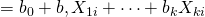Remember that point estimates do not carry a particular level of probability, or level of confidence, because points have no “width” above which there is an area to measure. This was why we developed confidence intervals for the mean and proportion earlier. The same concern arises here also. There are actually two different approaches to the issue of developing estimates of changes in the independent variable, or variables, on the dependent variable. The first approach wishes to measure the expected mean value of y from a specific change in the value of x: this specific value implies the expected value. Here the question is: what is the mean impact on y that would result from multiple hypothetical experiments on y at this specific value of x. Remember that there is a variance around the estimated parameter of x and thus each experiment will result in a bit of a different estimate of the predicted value of y.

The second approach to estimate the effect of a specific value of x on y treats the event as a single experiment: you choose x and multiply it times the coefficient and that provides a single estimate of y. Because this approach acts as if there were a single experiment the variance that exists in the parameter estimate is larger than the variance associated with the expected value approach.

The conclusion is that we have two different ways to predict the effect of values of the independent variable(s) on the dependent variable and thus we have two different intervals. Both are correct answers to the question being asked, but there are two different questions. To avoid confusion, the first case where we are asking for the expected value of the mean of the estimated y, is called a confidence interval as we have named this concept before. The second case, where we are asking for the estimate of the impact on the dependent variable y of a single experiment using a value of x, is called the prediction interval. The test statistics for these two interval measures within which the estimated value of y will fall are:

Confidence Interval for Expected Value of Mean Value of y for x=xp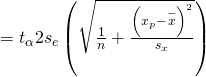Prediction Interval for an Individual y for x = xp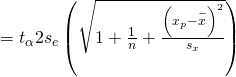Where se is the standard deviation of the error term and sx is the standard deviation of the x variable.

The mathematical computations of these two test statistics are complex. Various computer regression software packages provide programs within the regression functions to provide answers to inquires of estimated predicted values of y given various values chosen for the x variable(s). It is important to know just which interval is being tested in the computer package because the difference in the size of the standard deviations will change the size of the interval estimated. This is shown in (Figure).

Prediction and confidence intervals for regression equation; 95% confidence level.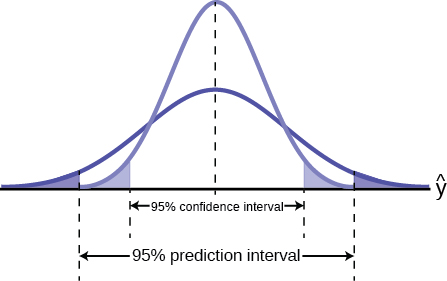(Figure) shows visually the difference the standard deviation makes in the size of the estimated intervals. The confidence interval, measuring the expected value of the dependent variable, is smaller than the prediction interval for the same level of confidence. The expected value method assumes that the experiment is conducted multiple times rather than just once as in the other method. The logic here is similar, although not identical, to that discussed when developing the relationship between the sample size and the confidence interval using the Central Limit Theorem. There, as the number of experiments increased, the distribution narrowed and the confidence interval became tighter around the expected value of the mean.

It is also important to note that the intervals around a point estimate are highly dependent upon the range of data used to estimate the equation regardless of which approach is being used for prediction. Remember that all regression equations go through the point of means, that is, the mean value of y and the mean values of all independent variables in the equation. As the value of x chosen to estimate the associated value of y is further from the point of means the width of the estimated interval around the point estimate increases. Choosing values of x beyond the range of the data used to estimate the equation possess even greater danger of creating estimates with little use; very large intervals, and risk of error. (Figure) shows this relationship.

Confidence interval for an individual value of x, Xp, at 95% level of confidence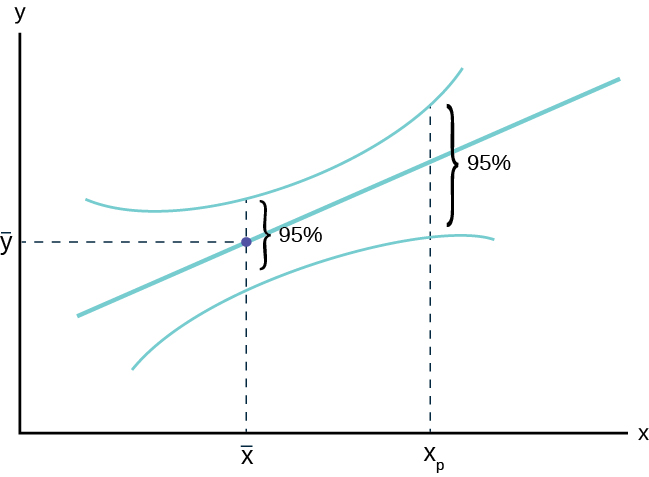(Figure) demonstrates the concern for the quality of the estimated interval whether it is a prediction interval or a confidence interval. As the value chosen to predict y, Xp in the graph, is further from the central weight of the data,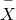, we see the interval expand in width even while holding constant the level of confidence. This shows that the precision of any estimate will diminish as one tries to predict beyond the largest weight of the data and most certainly will degrade rapidly for predictions beyond the range of the data. Unfortunately, this is just where most predictions are desired. They can be made, but the width of the confidence interval may be so large as to render the prediction useless. Only actual calculation and the particular application can determine this, however.

Recall the third exam/final exam example .

We found the equation of the best-fit line for the final exam grade as a function of the grade on the third-exam. We can now use the least-squares regression line for prediction. Assume the coefficient for X was determined to be significantly different from zero.

Suppose you want to estimate, or predict, the mean final exam score of statistics students who received 73 on the third exam. The exam scores (x-values) range from 65 to 75. Since 73 is between the x-values 65 and 75, we feel comfortable to substitute x = 73 into the equation. Then: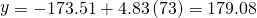We predict that statistics students who earn a grade of 73 on the third exam will earn a grade of 179.08 on the final exam, on average.

a. What would you predict the final exam score to be for a student who scored a 66 on the third exam?

a. 145.27

b. What would you predict the final exam score to be for a student who scored a 90 on the third exam?

b. The x values in the data are between 65 and 75. Ninety is outside of the domain of the observed x values in the data (independent variable), so you cannot reliably predict the final exam score for this student. (Even though it is possible to enter 90 into the equation for x and calculate a corresponding y value, the y value that you get will have a confidence interval that may not be meaningful.)

To understand really how unreliable the prediction can be outside of the observed x values observed in the data, make the substitution x = 90 into the equation.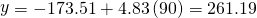The final-exam score is predicted to be 261.19. The largest the final-exam score can be is 200.

True or False? If False, correct it: Suppose you are performing a simple linear regression of Y on X and you test the hypothesis that the slope β is zero against a two-sided alternative. You have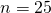observations and your computed test (t) statistic is 2.6. Then your P-value is given by .01 < P < .02, which gives borderline significance (i.e. you would reject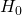at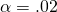but fail to rejectat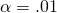).

True.

t(critical, df = 23, two-tailed, α = .02) = ± 2.5

tcritical, df = 23, two-tailed, α = .01 = ± 2.8

An economist is interested in the possible influence of “Miracle Wheat” on the average yield of wheat in a district. To do so he fits a linear regression of average yield per year against year after introduction of “Miracle Wheat” for a ten year period.

The fitted trend line is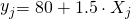(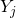: Average yield in j year after introduction)

(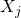: j year after introduction).

1. What is the estimated average yield for the fourth year after introduction?
2. Do you want to use this trend line to estimate yield for, say, 20 years after introduction? Why? What would your estimate be?
1.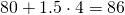2. No. Most business statisticians would not want to extrapolate that far. If someone did, the estimate would be 110, but some other factors probably come into play with 20 years.

An interpretation of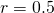is that the following part of the Y-variation is associated with which variation in X:

1. most
2. half
3. very little
4. one quarter
5. none of these

d. one quarter

Which of the following values of r indicates the most accurate prediction of one variable from another?

1.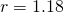2.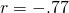3.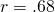b.